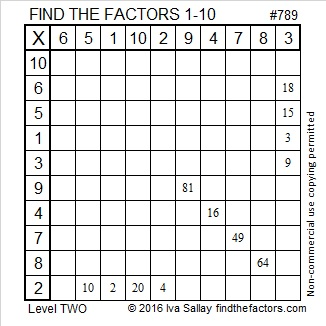# 789 and Level 2

789 consists of exactly three consecutive numbers so it is divisible by 3.Print the puzzles or type the solution on this excel file: 10-factors-788-794

————————————–

• 789 is a composite number.
• Prime factorization: 789 = 3 x 263
• The exponents in the prime factorization are 1 and 1. Adding one to each and multiplying we get (1 + 1)(1 + 1) = 2 x 2 = 4. Therefore 789 has exactly 4 factors.
• Factors of 789: 1, 3, 263, 789
• Factor pairs: 789 = 1 x 789 or 3 x 263
• 789 has no square factors that allow its square root to be simplified. √789 ≈ 28.08914.789 is the sum of consecutive prime numbers 2 different ways:

• 257 + 263 + 269 = 789 (that’s 3 consecutive primes.)
• 3 + 5 + 7 + 11 + 13 + 17 + 19 + 23 + 29 + 31 + 37 + 41 + 43 + 47 + 53 + 59 + 61 + 67 + 71 + 73 + 79 = 789 (that’s 21 consecutive primes!)

789 is the sum of three squares eight different ways:

• 28² + 2² + 1² = 789
• 26² + 8² + 7² = 789
• 25² + 10² + 8² = 789
• 23² + 16² + 2² = 789
• 23² + 14² + 8² = 789
• 22² + 17² + 4² = 789
• 22² + 16² + 7² = 789
• 20² + 17² + 10² = 789